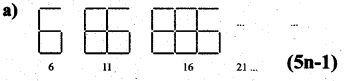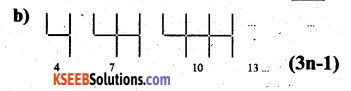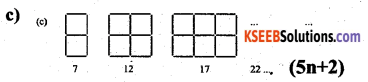# KSEEB Solutions for Class 7 Maths Chapter 12 Algebraic Expressions Ex 12.4

Students can Download Chapter 12 Algebraic Expressions Ex 12.4, Question and Answers, Notes Pdf, KSEEB Solutions for Class 7 Maths, Karnataka State Board Solutions help you to revise complete Syllabus and score more marks in your examinations.

## Karnataka State Syllabus Class 7 Maths Chapter 12 Algebraic Expressions Ex 12.4

Question 1.
Observe the patterns of digits made from line segments of equal length. You will find such segmented digits on the display of electronic watches or calculators.
If the number of digits framed is taken to be n, the number of segments required to form n digits is given by the algebraic expression appearing on the right of each pattern.
How many segments are required to form 5, 10, 100 digits of the kind 6, 4, 8.Solution:
i) The number of segments required to form
5 digits of this kind
5n + 1 = 5 × 5 + 1 = 25 + 1 = 26

ii) The number of segments required to form 10 digits of this kind
5n + 1 = 5 × 10 + 1 = 50 + 1 = 51

iii) The number of segments required to form 100 digits of this kind
5n + 1 = 5 × 100 + 1 = 501Let the number of digits formed be ‘n’.
Therefore the number of segments required to form ‘n’ digits is given by the algebraic expression 3n + 1.
i) The number of segments required to form 5 digits of this kind.
3n + 1 = 3 × 5 + 1 = 15 + 1 = 16

ii) The number of segments required to form 10 digits of this kind
3n + 1 = 3 × 10 + 1 = 30 + 1 = 31

iii) The number of segments required to form 100 digits of this kind
3n + 1 = 3 × 100 + 1 = 300 + 1 = 301Let the number of digits formed be ‘n’. Therefore the number of segments required to form ‘n’ digits is given by the algebraic expression 5n + 2.
i) The number of segments required to form 5 digits of this kind.
5n + 2 = 5 × 5 + 2 = 25 + 2 = 27
ii) The number of segments required to form 10 digits of this kind
5n + 2 = 5 × 10 + 2 = 50 + 2 = 52iii) The number of segments required to form 100 digits of this kind
5n + 2 = 5 × 100 + 2 = 500 + 2 = 502

Question 2.
Use the given algebraic expression to complete the table of number patterns.
Solution:error: Content is protected !!